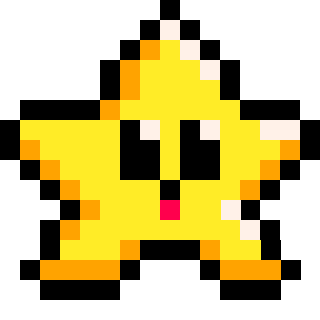# Create_tables

##### Empty Table

To create an empty table, use the opening and closing curly braces `{}`, like this:

``mytable = {}``
mytable

This is useful for preparing a variable to hold the table in your `_init()` function during set up. Then use `add()` to insert data into the table later.##### List

To create a list type of table, you can write the values inside the curly braces, separated by commas, and let the table automatically number them.

``mytable = { "superman", "spiderman", "ironman" }``
mytable
1 superman
2 spiderman
3 ironman

You can also number them yourself, if you need to (and order them yourself):

``mytable = { ="kirk", `="spock", ="scotty" }`
mytable
1 spock
2 kirk
3 scotty

Or you can add numbered keys to any table already created by using square brackets `[]` after the table name, `mytable[key]`, like this:

``````mytable = {}  --empty table
mytable = "picard"
mytable = "laforge"
mytable = "worf"``````
mytable
1 picard
2 laforge
3 worf##### Object

To create an object type of table, you can name the keys and use any data type as the value; even other tables or functions.

``mytable = { sprite=1, x=10, y=5, state="run" }``
mytable
sprite 1
x 10
y 5
state run

Another way to write this is vertically, so that it is more readable for more complex object tables:

``````mytable = {
sprite = 1,
x = 10,
y = 5,
state = "run"
}``````

When writing your table entries vertically like this, make sure you still separate each entry with a comma. Forgetting a comma is often the cause of the "`unlcosed {`" error.

Another way to write this type of table vertically, that some people prefer, is to create the empty object table first, then insert each entry separately by using square brackets `[]` after the table name, `mytable[key]`, like this:

``````mytable = {}
mytable[sprite] = 1
mytable[x] = 10
mytable[y] = 5
mytable[state] = "run"
``````

Notice that this way does not require commas and is less likely to cause confusing errors, however it costs more tokens and characters. So if you already know what should be inside the table, it is better to insert the entries at the same time as creating the table.##### Collection of Objects

To create a collection type of table that holds multiple objects, you will want to use nested tables. (What is a Nested Table?)

``````mytable= { --open outer table
{} --inner table
} --close outer table``````
mytable
1
(inner table)

Nested tables can be hard to wrap your head around at first, but the more you work with them, the easier and more logical they will be to use. Here is what a single object table inside of a collection looks like, but they become more powerful and useful with more objects you store in the collection.

``````items= {
{ name="sword", attack="5" },  --inner table 1
{ name="sheild", armor="10" }  --inner table 2
}``````
items
1
 name sword attack 5
2
 name sheild armor 10

A collection of objects is a great way to create, store, and control multiple objects in your game such as bullets, items, enemies, and particles.

#### Collection of Bullets:

```bullets={
{ x=65, y=51 },
{ x=22, y=25 },
{ x=33, y=15 }
}```

#### Collection of Items:

```coins={
{ sprite=1, x=10, y=5, name="copper" },
{ sprite=2, x=20, y=25, name="silver" },
{ sprite=3, x=30, y=15, name="gold" }
}```

#### Collection of Enemies:

```enemies={
{ sprite=10, x=30, y=15, name="goomba" },
{ sprite=11, x=40, y=35, name="koopa" },
{ sprite=12, x=50, y=65, name="boo" }
}```

These collections of objects can be easily updated and drawn in your game using loops.##### Classes and Instances

In Object-Oriented Programming, the term class refers to a blueprint you use to create multiple objects from. So you can create a blueprint enemy table like this:

``````enemy = {
type = 0,
sp = 1,
x = 0,
y = 0,
dx = 0,
dy = 0,
function update(self)
self.x += self.dx
self.y += self.dy
end,
function draw(self)
spr(self.sp, self.x, self.y)
end
}``````

This class can be copied whenever you want to create more enemy objects and adjust the starting variables for different types of enemies.

``````goblin = enemy --copy enemy class
goblin.type = 3
goblin.x = 6
goblin.y = 10

ogre = enemy  --copy enemy class
ogre.type = 4
ogre.x = 40
ogre.y = 50``````

This example is just to show how `goblin` and `ogre` both copy the `enemy` table which remains unchanged and is used as the template or blueprint for both types of enemies. The rest of the variables and functions from the enemy table are also copied.

enemy
type 0
x 0
y 0

goblin
type 3
x 6
y 10

#### Instance

ogre
type 4
x 40
y 50

Whenever you copy a class to create an object, that new object is referred to as an instance. So, in PICO-8 these are all tables...

Object is a table that holds all data and even functions dealing with one specific entity in your game.
Class is a table that is used as a template or blueprint for creating similar objects.
Instance is a table (exact same as an object) that was created by copying a class.#### Shorthand

You can use the shorthand` .` to refer to a table key instead of `[]`, like this:

#### mytable[key]

``````mytable = {}
mytable[sprite] = 1
mytable[x] = 10
mytable[y] = 5
mytable[state] = "run"
``````

#### mytable.key

``````mytable = {}
mytable.sprite = 1
mytable.x = 10
mytable.y = 5
mytable.state = "run"
``````

These two can also be used together to access inner table keys of nested tables, useful when you have a collection of objects (described above).

``enemies.sprite``

This will look at the enemies table, then find the first entry `` inside of that, then it will look inside of that inner table and find the `sprite` key, and get the value stored there.576

22 Nov 2023

`Font`